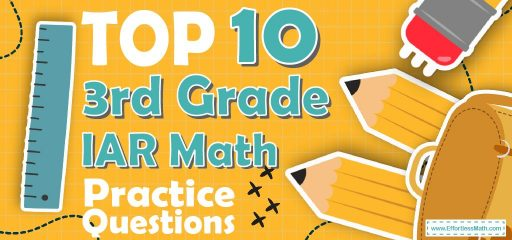# Top 10 3rd Grade IAR Math Practice QuestionsIf your 3rd-grade student has reviewed the 3rd Grade IAR Math materials and has a relative mastery of all math topics, we suggest solving the Top 10 3rd-grade IAR Math Practice Questions.

In fact, using the Top 10 3rd-Grade IAR Math Practice Questions, the most important mathematical concepts of the 3rd Grade IAR exam are reviewed and the strengths and weaknesses of your 3rd-grade student are identified.

The top 10 3rd Grade IAR Math Practice Questions have been updated in accordance with the 2022 3rd Grade IAR Math exam guidelines. The full answer and explanation are also provided at the end of the post.

The Absolute Best Book to Ace the 3rd Grade IAR Math Test

## 3rd Grade IAR Math Practice Questions

1- There are $$6$$ numbers in the box below. Which of the following list shows only odd numbers from the numbers in the box?

A. $$15, 29, 42$$

B. $$15, 29, 83$$

C. $$15, 30, 42$$

D. $$42, 18, 30$$

2- Mia’s goal is to save $$140$$ to purchase her favorite bike.
In January, she saved $$36$$.
In February, she saved $$28$$.
How much money does Mia need to save in March to be able to purchase her favorite bike?

A. $$28$$

B. $$30$$

C. $$52$$

D. $$76$$

3- Michelle has $$72$$ old books. She plans to send all of them to the library in their area. If she puts the books in boxes that can hold $$9$$ books, which of the following equations can be used to find the number of boxes she will use?

A. $$72 + 9 =$$ ______

B. $$72 × 9 =$$ ______

C. $$72 – 9 =$$ ______

D. $$72 ÷ 9 =$$ ______

4- Eva had $$983$$ cards. Then, she gave $$349$$ of the cards to her friend Alice. After that, Eva lost $$260$$ cards.
Which equation can be used to find the number of cards Eve has now?

A. $$983 – 349 + 260 =$$ _____

B. $$983 – 349 –260 =$$ _____

C. $$983 +349 +260 =$$ _____

D. $$983 +349 –260 =$$ _____

5- The length of the following rectangle is $$7$$ centimeters and its width is $$3$$ centimeters. What is the area of the rectangle?

A. $$10\space cm^2$$

B. $$20\space cm^2$$

C. $$14\space cm^2$$

D. $$21\space cm^2$$

6- Which number is made up of $$4$$ hundred, $$8$$ tens, and $$4$$ ones?

A. $$4,084$$

B. $$484$$

C. $$448$$

D. $$844$$

7- Look at the spinner above. On which color is the spinner most likely to land?

A. RED

B. GREEN

C. YELLOW

D. NONE

8- A group of third-grade students recorded the following distances that they jumped.

A. $$23$$

B. $$24$$

C. $$32$$

D. $$34$$

9- Emma flew $$2,448$$ miles from Los Angeles to New York City. What is the number of miles Emma flew rounded to the nearest thousand?

A. $$2,000$$

B. $$2,400$$

C. $$2,500$$

D. $$3,000$$

10- A number sentence such as $$31 + Z = 98$$can be called an equation. If this equation is true, then which of the following equations is not true?

A. $$98 – 31 = Z$$

B. $$98 – Z= 31$$

C. $$Z– 31 = 98$$

D. $$Z+31 = 98$$

Best 3rd Grade IAR Math Prep Resource

1- B
An easy way to tell whether a large number is odd or even is to look at its final digit. If the number ends with an odd digit $$(1, 3, 5, 7, or\space 9)$$, then it’s odd. On the other hand, if the number ends with an even digit or $$0 (0, 2, 4, 6\space or\space 8)$$, then it is even.
$$15, 29,$$ and $$83$$ end with odd digits, so they are odd numbers.

2- D
She saves $$36$$ and $$28$$ so she has $$64$$.
$$140 – 64 = 76$$ she needs to save.

3- D
Michelle divides $$72$$ books into $$9$$ boxes therefore $$72 ÷ 9$$ formula is correct.

4- B
Eva gave $$349$$ of her $$983$$ cards to her friend so her cards are $$983-349$$, then she lost $$260$$ cards so now she has $$983-349-260=374$$ cards.

5- D
Use the formula of a rectangle:
$$Area= length × width$$
Area $$=3cm×7cm =21cm^2$$

6- B
Add $$4$$ hundred and $$8$$ tens and $$4$$ ones
So we have :$$400 + 80 + 4 = 484$$

7- A
The chances of landing on red are $$3$$ in $$6$$
The chances of landing on yellow are $$1$$ in $$6$$
The chances of landing on the green are $$2$$ in $$6$$
The chances of landing on red are more than in other colors.

8- C
$$32$$ inches distance most occur in this table.

9- A

10- C
All these equations are true:
$$98 – 31 = Z$$
$$98 – Z = 31$$
$$Z + 31 = 98$$

The Best Books to Ace the 3rd Grade IAR Math Test

### What people say about "Top 10 3rd Grade IAR Math Practice Questions - Effortless Math: We Help Students Learn to LOVE Mathematics"?

No one replied yet.

X
27% OFF

Limited time only!

Save Over 27%

SAVE $5 It was$18.99 now it is \$13.99